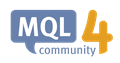# still having Constant Expression Required

This error shows up when i try to find max array from these variables: Constant Expression Required. i've had a go, any ideas?

``` double num_array;
num_array= RFTPD8;
num_array= RFTPD7;
num_array= RFS3;

double num_array= {RFTPD8,RFTPD7,RFS3};
int maxValueIdx=ArrayMaximum(num_array,WHOLE_ARRAY,0);```

123Yaj:

This error shows up when i try to find max array from these variables: Constant Expression Required. i've had a go, any ideas?

This line seems unnecessary - redefining the array.

```    double num_array= {RFTPD8,RFTPD7,RFS3};
```
Comment it out and try again

R4tna C #:

This line seems unnecessary - redefining the array.

Comment it out and try again
``` double num_array;
num_array= RFTPD8;
num_array= RFTPD7;
num_array= RFS3;

//    double num_array= {RFTPD8,RFTPD7,RFS3};
int maxValueIdx=ArrayMaximum(num_array,WHOLE_ARRAY,0);```
done, but the value is only the maximum of the number of values, no the maximum of the values itself. I am looking for the maximum value (RFS3).

123Yaj #: done, but the value is only the maximum of the number of values, no the maximum of the values itself. I am looking for the maximum value (RFS3).

You found the index in the array with the maximum value. Read the maximum value out of the array using the index.

123Yaj #:
done, but the value is only the maximum of the number of values, no the maximum of the values itself. I am looking for the maximum value (RFS3).

The documentation explains how the return value works

https://docs.mql4.com/array/arraymaximumArrayMaximum - Array Functions - MQL4 Reference
• docs.mql4.com
ArrayMaximum - Array Functions - MQL4 Reference

William Roeder #:

You found the index in the array with the maximum value. Read the maximum value out of the array using the index.

thanks, Maxindex[array];

How would i call the value below the maximum value. something like this?

``` double num_array;
num_array= RFTPD8;
num_array= RFTPD7;
num_array= RFS3;

//    double num_array= {RFTPD8,RFTPD7,RFS3};
int maxValueIdx=ArrayMaximum(num_array,WHOLE_ARRAY,0);

double   NA= maxValueIdx[num_array];

double secval = maxValueIdx-1;

double NA1 = SSecval[num_array];```

123Yaj #:

thanks, Maxindex[array];

How would i call the value below the maximum value. something like this?

The usage would be:

```int maxValueIdx=ArrayMaximum(num_array,WHOLE_ARRAY,0);

double   NA=num_array[maxValueIdx];

```

This next statement is not reliable - if the maxValueIdx is 0, you will get secVal = -1

```double secval = maxValueIdx-1;
```

That would result in an array out of bounds error should you try and access the array (program crash).

R4tna C #:

The usage would be:

This next statement is not reliable - if the maxValueIdx is 0, you will get secVal = -1

That would result in an array out of bounds error should you try and access the array (program crash).

c++ has something like this *???

```int secondLargest(int num_array[], int n=17)

for (int i = 0; i < n; i++)
{
if (num_array[i] != FD)
{
if (secondLargest == -1)
secondLargest = i;
else if (arr[i] > arr[secondLargest])
secondLargest = i;
}
}
return secondLargest;

int n = ArraySize(num_array)/ArraySize(num_array);
int second_largest = secondlargest(num_array,n);

double FD2= num_array[second_largest];
```

123Yaj #:

c++ has something like this *???

I am not sure about your code, but have you considered using the sorting function?

That way you will have the largest value at the end (assuming you use the default direction=MODE_ASCEND) and you can work backwards to get the 2nd largest

https://docs.mql4.com/array/arraysortArraySort - Array Functions - MQL4 Reference
• docs.mql4.com
ArraySort - Array Functions - MQL4 Reference